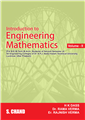Home > Tech Professional > Engineering > Introduction to Engineering Mathematics Volume-II (For APJAKTU, Lucknow)Introduction to Engineering Mathematics Volume-II (For APJAKTU, Lucknow), 9/e

H K Dass, Rajnish Verma & Dr. Rama Verma

 ISBN : 9789352835348 Pages : 576 Binding : Paperback Language : English Imprint : S. Chand Publishing Trim size : 6.75 X 9.5 inches Weight : 0.25kg © year : 2019
 List Price :425.00
 Offer Price :340.00

20% OFF

In Stock

Credit Card / Debit Card / Internet BankingIntroduction to Engineering Mathematics Volume-II has been thoroughly revised according to the New Syllabi (2018 onwards) of Dr. A.P.J. Abdul Kalam Technical University (AKTU, Lucknow). The book contains 15 chapters divided among five modules - Ordinary Differential Equations of Higher Order, Multivariable Calculus-II, Sequence and Series, Complex Variable Differentiation and Complex Variable-Integration. It contains numerous solved examples from question papers of examinations recently held by different universities and engineering colleges so that the students may not find any difficulty while answering these problems in their final examination. • Very short questions of 2 Marks have been added at the end of each chapters for better preparation of concepts. • Latest Solved Question Papers have been solved and included in the textbook. Module-I: Ordinary Differential Equations of Higher Order: 1. Linear Differential Equations of nth Order with Constant Coefficient, 2. Simultaneous Linear Differential Equations, 3. Second Order Linear Differential Equations, 4. Method of Variation of Parameters, 5. Series Solutions of Second Order Differential Equations Module-II: Multivariable Calculus-II: 6. Improper Integrals of Different Types, 7. Gamma, Beta Function, 8. Application of Definite Integration Module-III: Sequence and Series: 9. Sequence and Series, 10. Fourier Series Module-IV: Complex Variable Differentiation: 11. Functions of Complex Variable, Analytic Function, 12. Conformal Mapping Module-V: Complex Variable-Integration: 13. Complex Integration, 14. Taylor's and Laurent's Series, 15. The Calculus of Residues (Integration)More Books By AuthorIntroduction to Engineering ...

9789352834075425.00340.00

20% OFFEngineering Mathematics-I ...

9789352534616375.00300.00

20% OFF425.00340.00